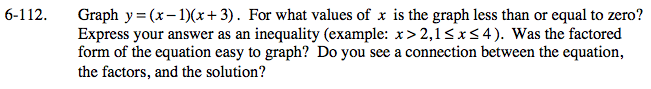### Home > PC > Chapter 6 > Lesson 6.3.1 > Problem6-112

6-112.

Graph y = (x − 1)(x + 3). For what values of x is the graph less than or equal to zero? Express your answer as an inequality (example: x > 2, 1 ≤ x ≤ 4). Was the factored form of the equation easy to graph? Do you see a connection between the equation, the factors, and the solution? Homework Help ✎Where is the curve below the x-axis?